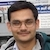# NAEST 2016 Screening Test: CalorimetryBy

A beaker is filled with water up to a certain height. The water temperature is measured with a laboratory thermometer. The beaker is kept on a heater for two minutes. The water temperature rises which is measured again. The students need to find the volume of the water from the marks on the beaker. Also, student needs to find the initial and final temperatures from the thermometer readings. Finally, the rate at which heat is supplied to the water is to be determined. The heat capacity of the water is given as 4200 J/kg°C.

Question: The specific heat capacity of water is 4200 J/kg-°C. The time duration of water heating is 2 minutes. Answer the following questions:

1. The volume of water = _______
2. The temperature of normal water =________
3. The temperature of water after heating =______
4. The rate at which heat was given to the water is around
1. 180 W
2. 160 W
3. 140 W
4. 120 W

Solution: The beaker is of 500 ml capacity. The water is filled up to the 100 ml mark. Thus, the volume of water is 100 ml or 100 cc.

The thermometer reading shows the initial temperature of the water as $T_i=22\,$ °C. Similary, the final temperature of the water is $T_f=68\,$ °C.

The density of water is 1 g/cc or 1000 kg/m3. Thus, the mass of 100 ml of water is \begin{align} m=\rho V=100\, \mathrm{g} = 0.1\,\mathrm{kg}. \end{align}

The heat energy required to raise the temperature of 100 g water from 22 °C to 68 °C is given by \begin{align} Q&=mS\Delta T=mS(T_f-T_i) \\ &=0.1\times 4200 \times (68-22) \\ &=420\times 46=19320\,\mathrm{J} \end{align}

Thus, 19320 J of energy is given to the water in 2 min (120 seconds). Hence, the rate at which heat is given to the water is \begin{align} P=\frac{Q}{t}=\frac{19320}{120}=161\,\mathrm{W}. \end{align} The option (B) is correct.